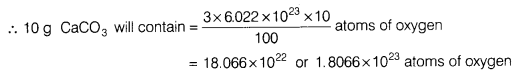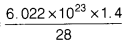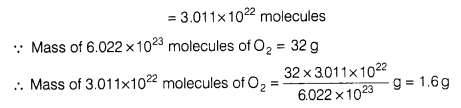# Calculate the number of oxygen atoms in 10 g

1. Calculate the number of oxygen atoms in 10 g of CaCO3.
[Atomic mass of Ca = 40 u, C = 12 u and 0=16 u]
2. Calculate the mass of O2 molecules which contain same number of molecules as 1.4 g of nitrogen (N2).
1. Molar mass of CaCO3 = Atomic mass of (Ca + C + 3 x O)
= 40 + 12 + 3 x 16=100 g
∵ 100g CaCO3 contains 3 x 6.022 x 1023 atoms of oxygen.2. Molar mass of N2 = 2 x 14 g = 28 g
Molar mass of O2 = 2 x 16 g = 32 g
∵ 28 g N2 contains 6.022 x 1023 molecules
∵ 1.4 g N2 will contain =molecules